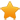Switch Editions?
CancelChannel: CodeSection,代码区,网络安全 - CodeSec
Viewing all articles

# Species richness analysis with R and sf

0
0

Este contenido está disponible en espaolaquí.

Counting how many underlying features intersect a layer of interest is a very common geoprocessing task, and has always been possible with GIS software. I’ve been getting into the sf package to do spatial analyses in R, and wanted to document and share this approach for quantifying and plotting species richness.

The code below is a fully reproducible (as long as the relevant packages are installed). I chose Costa Rica as an example, and rather than downloading and reading distribution data for actual species we will generate random points iteratively. Shapefiles can be read easily into sf using st_read . Here, I calculated gridded richness for point data first and then for convex hulls because those are some of the most common approaches used nowadays.

These are the main steps in the process:

Setup subset a world map to get a single-country polygon generate a random number of random points within the country for n different ‘species’ create smoothed convex hulls around each set of points Geoprocessing generate a grid to cover our country polygon intersect and join the multipoint feature set and the convex hulls with the grid (separately) Plotting plot the richness grids using perceptually-uniform and colorblind-friendly palettes using ggplot , scico and sf

The different spatial elements look like this:

The blank map

Randomly generated points for n ‘species’

Smoothed convex hulls around the sets of points

Grid for calculating richness

Gridded richness for points

Gridded richness for convex hulls

Notes:

The rerun function from purrr is awesome, I hadn’t seen it before but it is a very cool replacement for most basic for loops. I don’t know how I missed it.

When plotting, we use a bounding box and and the st_touches function with some tidyverse magic (slicing and plucking) to get the adjacent countries so we can add more context to our plot and focus in on our polygon of interest without having to set up the limits manually.

We counted the number of intersecting features using a grid, but this can also be done using political boundaries (states, municipalities, counties), vegetation types or any other layer of interest.

Any feedback is welcome.

R code:

# load packages library(sf) library(dplyr) library(ggplot2) library(scico) library(rnaturalearth) library(purrr) library(smoothr) # get a world map worldMap <- ne_countries(scale = "medium", type = "countries", returnclass = 'sf') # filter country CRpoly <- worldMap %>% filter(sovereignt=="Costa Rica") # generate random points, then name each list element sp_occ <- rerun(12,st_sample(CRpoly,sample(3:20,1))) names(sp_occ) <- paste0("sp_",letters[1:length(sp_occ)]) # to sf, with column of element names sflisss <- map(sp_occ,st_sf) %>% map2(.,names(.),~mutate(.x,id=.y)) #%>% sp_occ_sf <- sflisss %>% reduce(rbind) # to multipoint sp_occ_sf <- sp_occ_sf %>% group_by(id) %>% summarise() # set up bounds limsCR <- st_buffer(CRpoly,dist = 0.7) %>% st_bbox() # context adjacentPolys <- st_touches(CRpoly,worldMap) neighbours <- worldMap %>% slice(pluck(adjacentPolys,1)) # blank map divpolPlot <- ggplot()+ geom_sf(data=neighbours,color="white")+ geom_sf(data=CRpoly)+ coord_sf(xlim = c(limsCR["xmin"], limsCR["xmax"]), ylim = c(limsCR["ymin"], limsCR["ymax"]))+ scale_x_continuous(breaks = c(-84))+ theme(plot.background = element_rect(fill="#f1f2f3"), panel.background = element_rect(fill="#2F4051"), panel.grid = element_blank(), line = element_blank(), rect = element_blank()) # plot points spPointsPlot <- ggplot()+ geom_sf(data=neighbours,color="white")+ geom_sf(data=CRpoly)+ geom_sf(data=sp_occ_sf,aes(fill=id),pch=21)+ scale_fill_scico_d(palette = "davos",direction=-1,end=0.9,guide=FALSE)+ coord_sf(xlim = c(limsCR["xmin"], limsCR["xmax"]), ylim = c(limsCR["ymin"], limsCR["ymax"]))+ scale_x_continuous(breaks = c(-84))+ theme(plot.background = element_rect(fill="#f1f2f3"), panel.background = element_rect(fill="#2F4051"), panel.grid = element_blank(), line = element_blank(), rect = element_blank()) # smoothed convex hulls spEOOs <- st_convex_hull(sp_occ_sf) %>% smooth() # plot hulls hullsPlot <- ggplot()+ geom_sf(data=neighbours,color="white")+ geom_sf(data=CRpoly)+ geom_sf(data=spEOOs,aes(fill=id),alpha=0.7)+ scale_fill_scico_d(palette = "davos",direction=-1,end=0.9,guide=FALSE)+ coord_sf(xlim = c(limsCR["xmin"], limsCR["xmax"]), ylim = c(limsCR["ymin"], limsCR["ymax"]))+ scale_x_continuous(breaks = c(-84))+ theme(plot.background = element_rect(fill="#f1f2f3"), panel.background = element_rect(fill="#2F4051"), panel.grid = element_blank(), line = element_blank(), rect = element_blank()) # grid CRGrid <- CRpoly %>% st_make_grid(cellsize = 0.2) %>% st_intersection(CRpoly) %>% st_cast("MULTIPOLYGON") %>% st_sf() %>% mutate(cellid = row_number()) # calculate n per grid square for points richness_grid <- CRGrid %>% st_join(sp_occ_sf) %>% group_by(cellid) %>% summarize(num_species = n()) # calculate n per grid square for hulls richness_gridEOO <- CRGrid %>% st_join(spEOOs) %>% group_by(cellid) %>% summarize(num_species = n()) # blank grid gridPlot <- ggplot()+ geom_sf(data=neighbours,color="white")+ geom_sf(data=CRpoly)+ geom_sf(data=CRGrid)+ coord_sf(xlim = c(limsCR["xmin"], limsCR["xmax"]), ylim = c(limsCR["ymin"], limsCR["ymax"]))+ scale_x_continuous(breaks = c(-84))+ theme(plot.background = element_rect(fill="#f1f2f3"), panel.background = element_rect(fill="#2F4051"), panel.grid = element_blank(), line = element_blank(), rect = element_blank()) # plot gridded richness gridRichCR <- ggplot(richness_grid)+ geom_sf(data=neighbours,color="white")+ geom_sf(data=CRpoly,fill="grey",size=0.1)+ geom_sf(aes(fill=num_species),color=NA)+ scale_fill_scico(palette = "davos",direction=-1,end=0.9)+ coord_sf(xlim = c(limsCR["xmin"], limsCR["xmax"]), ylim = c(limsCR["ymin"], limsCR["ymax"]))+ scale_x_continuous(breaks = c(-84))+ theme(plot.background = element_rect(fill="#f1f2f3"), panel.background = element_rect(fill="#2F4051"), panel.grid = element_blank(), line = element_blank(), rect = element_blank())+labs(fill="richness") # richness based on hulls gridRichCR_eoo <- ggplot(richness_gridEOO)+ geom_sf(data=neighbours,color="white")+ geom_sf(data=CRpoly,fill="grey",size=0.1)+ geom_sf(aes(fill=num_species),color=NA)+ scale_fill_scico(palette = "davos",direction=-1,end=0.9)+ coord_sf(xlim = c(limsCR["xmin"], limsCR["xmax"]), ylim = c(limsCR["ymin"], limsCR["ymax"]))+ scale_x_continuous(breaks = c(-84))+ theme(plot.background = element_rect(fill="#f1f2f3"), panel.background = element_rect(fill="#2F4051"), panel.grid = element_blank(), line = element_blank(), rect =

Viewing all articles

### 《科學少年》柔珠海洋

More Pages to Explore .....